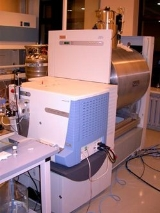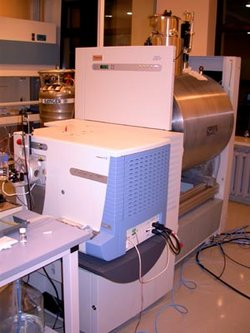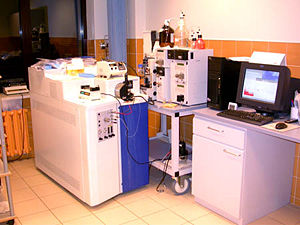xHybrid mass spectrometerEncyclopediaA hybrid mass spectrometer is a device for tandem mass spectrometry
Tandem mass spectrometry
Tandem mass spectrometry, also known as MS/MS or MS2, involves multiple steps of mass spectrometry selection, with some form of fragmentation occurring in between the stages.-Tandem MS instruments:...

that consists of a combination of two or more m/z separation devices of different types.

## Notation

The different m/z separation elements of a hybrid mass spectrometer can be represented by a shorthand notation. The symbol Q represents a quadrupole mass analyzer
The quadrupole mass analyzer is one type of mass analyzer used in mass spectrometry. As the name implies, it consists of 4 circular rods, set parallel to each other. In a quadrupole mass spectrometer the quadrupole is the component of the instrument responsible for filtering sample ions, based on...

, q is a radio frequency
Radio frequency is a rate of oscillation in the range of about 3 kHz to 300 GHz, which corresponds to the frequency of radio waves, and the alternating currents which carry radio signals...

collision quadrupole, TOF is a time-of-flight mass spectrometer, B is a magnetic sector and E is an electric sector.

A sector instrument
Sector instrument
A sector instrument is a general term for a class of mass spectrometer that uses a static electric or magnetic sector or some combination of the two as a mass analyzer. A popular combination of these sectors has been the BEB...

The quadrupole mass analyzer is one type of mass analyzer used in mass spectrometry. As the name implies, it consists of 4 circular rods, set parallel to each other. In a quadrupole mass spectrometer the quadrupole is the component of the instrument responsible for filtering sample ions, based on...

to form a hybrid instrument. A BEqQ configuration with a magnetic sector (B), electric sector (E), collision quadrupole (q) and m/z selection quadrupole (Q) have been constructed and an instrument with two electric sectors (BEEQ) has been described.A triple quadrupole mass spectrometer is a tandem mass spectrometer consisting of two quadrupole mass spectrometers in series, with a radio frequency only quadrupole between them to act as a collision cell for collision-induced dissociation...

with the final quadrupole replaced by a time-of-flight device is known as a quadrupole time-of-flight instrument. Such an instrument can be represented as QqTOF.

## Ion trap time-of-flight

In an ion trap instrument, ions are trapped in a quadrupole ion trap
A quadrupole ion trap exists in both linear and 3D varieties and refers to an ion trap that uses constant DC and radio frequency oscillating AC electric fields to trap ions. It is commonly used as a component of a mass spectrometer...

and then injected into the TOF. The trap can be 3-D or a linear trap.

## Linear ion trap and Fourier transform mass analyzers

A linear ion trap combined with a Fourier transform ion cyclotron resonance
Fourier transform ion cyclotron resonance
Fourier transform ion cyclotron resonance mass spectrometry, also known as Fourier transform mass spectrometry, is a type of mass analyzer for determining the mass-to-charge ratio of ions based on the cyclotron frequency of the ions in a fixed magnetic field...

or orbitrap
Orbitrap
An orbitrap is a type of mass spectrometer invented by Alexander Makarov. It consists of an outer barrel-like electrode and a coaxial inner spindle-like electrode that form an electrostatic field with quadro-logarithmic potential distribution....

mass spectrometer is marketed by Thermo Scientific as the LTQ FT and LTQ Orbitrap, respectively.